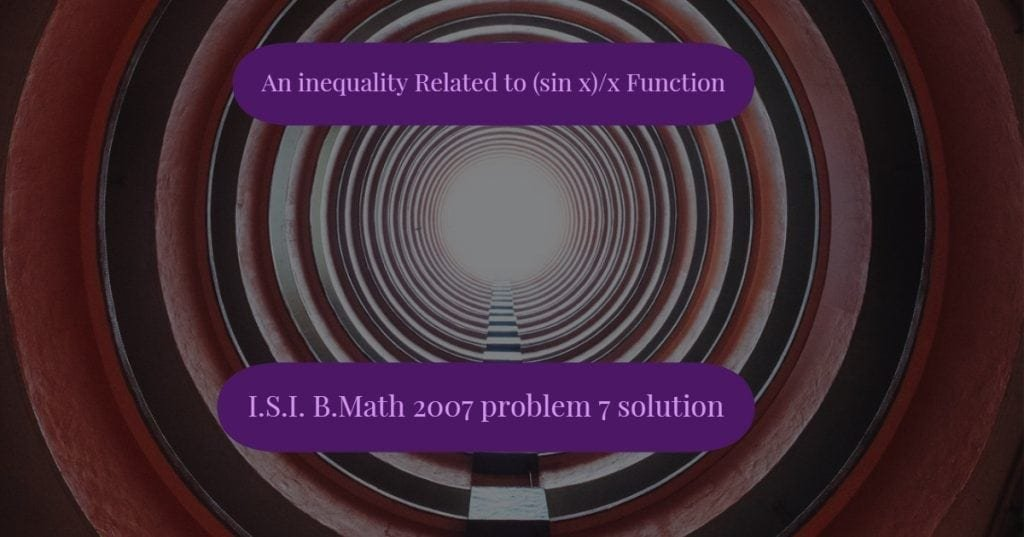How Cheenta works to ensure student success?
Explore the Back-Story

# An inequality related to (sin x)/x function | ISI BMath 2007This is a problem number 7 from ISI B.Math 2007 based on an inequality related to (sin x)/x function. Try out this problem.

Problem: An inequality related to (sin x)/x function

Let $\mathbf{0\leq \theta\leq \frac{\pi}{2}}$ . Prove that $\mathbf{\sin \theta \geq \frac{2\theta}{\pi}}$.

Discussion:

We consider the function $\mathbf{ f(x) = \frac{\sin x }{x} }$. The first derivative of this function is $\mathbf{ f'(x) = \frac{x \cos x - \sin x} {x^2} }$ In the interval $\mathbf{[0, \frac{\pi}{2}]}$ the numerator is always negative as x is less than tan x.

Hence f(x) is a monotonically decreasing function in the given interval. Hence f(x) attains least value at $\mathbf{x = \frac{\pi}{2} }$ which equals $\mathbf{ \frac{\sin\frac{\pi}{2}}{\frac{\pi}{2}} = \frac {2}{\pi}}$

Therefore $\mathbf{\frac{\sin \theta}{\theta} \ge \frac{2}{\pi}}$ in the given interval.

Our ISI CMI Program

How to use invariance in Combinatorics – ISI Entrance Problem – Video

This is a problem number 7 from ISI B.Math 2007 based on an inequality related to (sin x)/x function. Try out this problem.

Problem: An inequality related to (sin x)/x function

Let $\mathbf{0\leq \theta\leq \frac{\pi}{2}}$ . Prove that $\mathbf{\sin \theta \geq \frac{2\theta}{\pi}}$.

Discussion:

We consider the function $\mathbf{ f(x) = \frac{\sin x }{x} }$. The first derivative of this function is $\mathbf{ f'(x) = \frac{x \cos x - \sin x} {x^2} }$ In the interval $\mathbf{[0, \frac{\pi}{2}]}$ the numerator is always negative as x is less than tan x.

Hence f(x) is a monotonically decreasing function in the given interval. Hence f(x) attains least value at $\mathbf{x = \frac{\pi}{2} }$ which equals $\mathbf{ \frac{\sin\frac{\pi}{2}}{\frac{\pi}{2}} = \frac {2}{\pi}}$

Therefore $\mathbf{\frac{\sin \theta}{\theta} \ge \frac{2}{\pi}}$ in the given interval.

Our ISI CMI Program

How to use invariance in Combinatorics – ISI Entrance Problem – Video

This site uses Akismet to reduce spam. Learn how your comment data is processed.

### One comment on “An inequality related to (sin x)/x function | ISI BMath 2007”

1. […] Let . Prove that . Solution […]

### Knowledge Partner